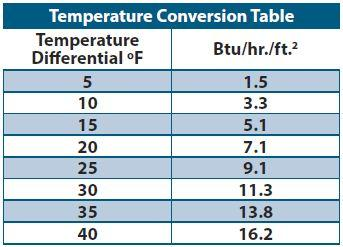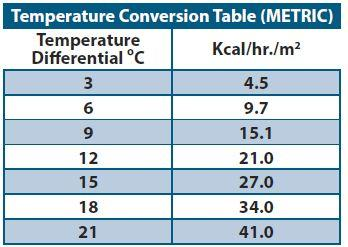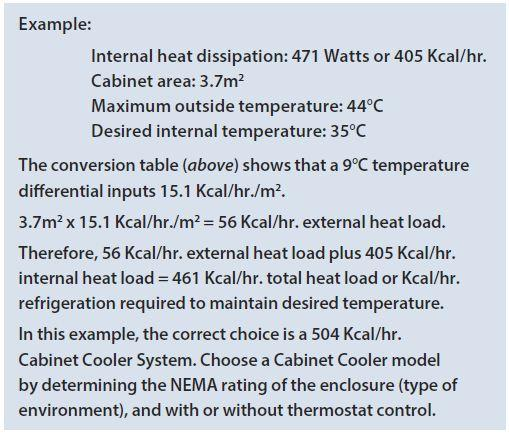# Sizing Guide - How to Calculate Heat Load for your Enclosure

To determine the correct model for your application, it is first necessary to determine the total heat load to which the control panel is subjected. This total heat load is the combination of two factors — heat dissipated within the enclosure and heat transfer from outside into the enclosure.

### To Calculate Btu/hr.:

1. First, determine the approximate Watts of heat generated within the enclosure. Watts x 3.41 = Btu/hr.

2. Then, calculate outside heat transfer as follows:
a. Determine the area in square feet exposed to the air, ignoring the top of the cabinet.
b. Determine the temperature differential between maximum surrounding temperature and desired internal temperature. Then, using the Temperature Conversion Table (below), determine the Btu/hr./ft.2 for that differential. Multiplying the cabinet surface area times Btu/hr./ft.2 provides external heat transfer in Btu/hr.

3. Add internal and external heat loads for total heat load.### To Calculate Kcal/hr.:

1. First, determine the approximate Watts of heat generated within the enclosure. Watts x .86 = Kcal/hr.
2. Then, calculate outside heat transfer as follows:
a. Determine the area in square meters exposed to the air, ignoring the top of the cabinet.
b. Determine the temperature differential between maximum surrounding temperature and desired internal temperature. Then, using the Metric Temperature Conversion Table (below), determine the Kcal/hr./m2 for that differential. Multiplying the cabinet surface area times Kcal/hr./m2 provides external heat transfer in Kcal/hr.
3. Add internal and external heat loads for total heat load.Vivek Engineers #22, 1st Floor, 1st Cross, Adj. to IIMB Compound, Bilekahalli Indl. Area, Bannerghatta Road, Bangalore - 560 076. Ph : 080 - 2648 1309, 4170 1145 Mob : 097404 39220 E- mail : sales@vivekengineers.net , durga@vivekengineers.net © Vivek Engineers. All Rights Reserved.